library(hbm4ecology)
library(rjags)
library(posterior)
library(tidyverse)
library(bayesplot)
library(GGally)
library(ggExtra)

The model rely on successive discrete (Binomial) distribution which the size parameter can get very low. This renders Normal approximation unreliable. Using Stan with Hamiltonian Monte Carlo would require to marginalize over the successive Binomial distribution. This requires some burdensome calculus. Hence we will use jags with a standard MCMC.

## Data and motivation

We turn to the successive removal Salmon data from the Nivelle, a 40-km river flowing from the Spanish Pyren'{e}es to the bay of Saint Jean de Luz. Details about the fishing procedure can be found in Chapter 4.2 of Parent and Prévost (2013) (page 83). We work with a complete dataset involving 3 years (2003-2005) with 11 sites located from the Nivelle River mouth to impassable upstream dams on the main stream and one of its tributaries. For each of the 33 experiments the number of fish caught were recorded for the first ($$C^{1}$$), second ($$C^{2})$$ and eventually third pass $$(C^{3})$$ of the electrofishing experiment. In addition, we provide the area $$S$$ in $$m^{2}$$ that was fished each time.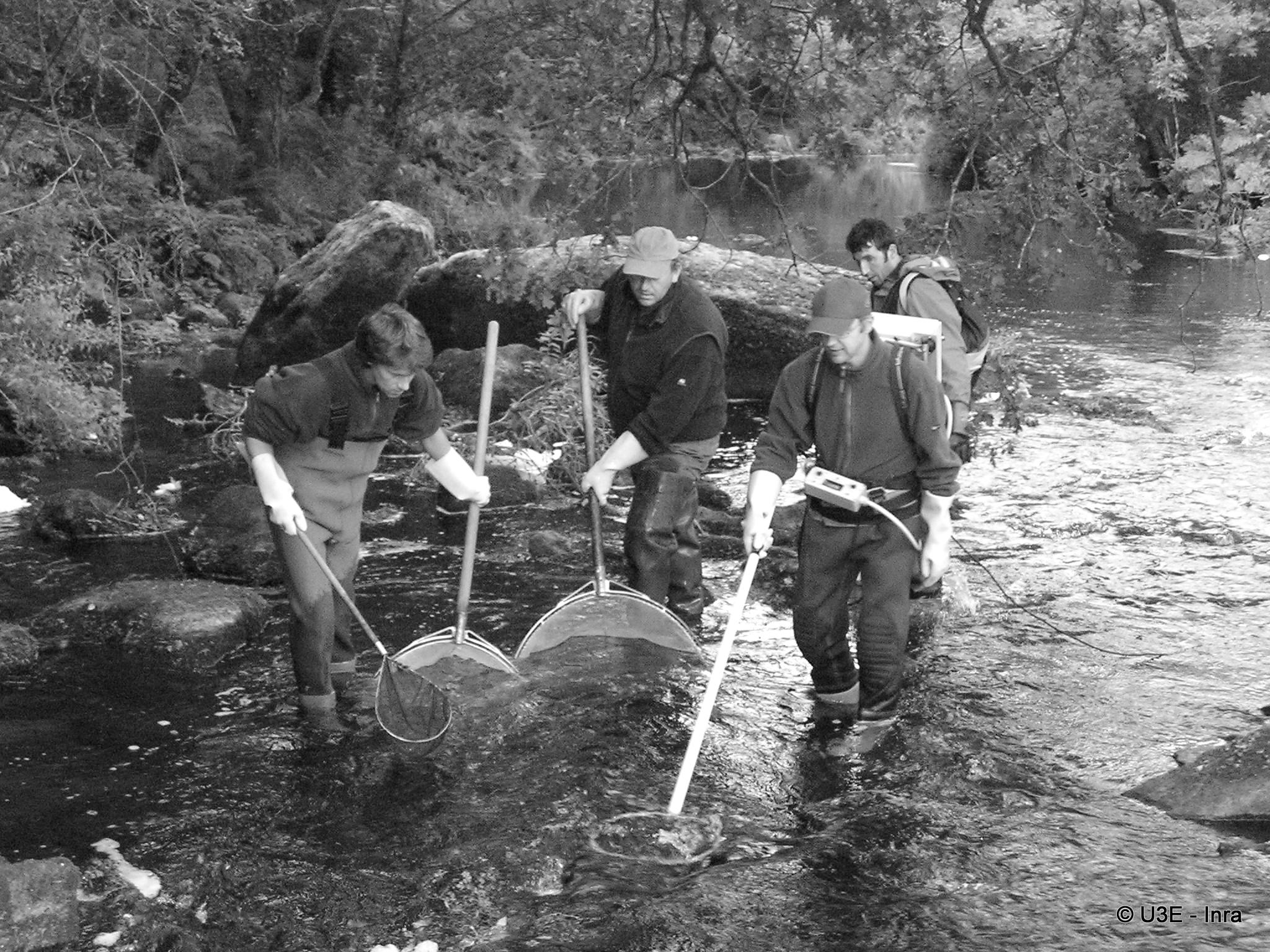Electrofishing of 0+ A. salmon juveniles

data("SucRemNivelle")
tibble(Site = seq(1,33)) %>%
bind_cols(bind_rows(SucRemNivelle[2:5])) %>%
knitr::kable(caption = "Three passes during successive removal electrofishing
on the Nivelle River (11 sites, years 2003-2004; NA = Not Available")
Three passes during successive removal electrofishing on the Nivelle River (11 sites, years 2003-2004; NA = Not Available
Site C1 C2 C3 S
1 23 14 3 608
2 35 7 NA 294
3 38 17 NA 565
4 41 28 15 564
5 17 8 NA 229
6 24 11 NA 200
7 63 21 NA 693
8 101 25 NA 571
9 78 17 NA 950
10 21 9 NA 430
11 51 16 NA 636
12 14 5 2 375
13 19 1 NA 441
14 14 7 2 590
15 37 4 NA 665
16 22 15 2 228
17 27 5 NA 236
18 53 8 4 599
19 32 4 NA 547
20 35 2 NA 870
21 37 7 NA 302
22 26 8 NA 611
23 24 16 4 413
24 36 12 NA 473
25 27 26 5 591
26 142 81 NA 1095
27 55 5 NA 254
28 22 2 NA 288
29 73 10 NA 667
30 104 28 NA 606
31 190 43 NA 712
32 16 2 NA 341
33 17 4 NA 632

## Model with independence between sites

The sampling distribution of the catch data are for each site $$i$$:

• $$C^1_i \sim Binomial(\nu_i, \pi_i)$$
• $$C^2_i \sim Binomial(\nu_i - C^1_i, \pi_i)$$
• $$C^3_i \sim Binomial(\nu_i - (C^1_i+C^2_i), \pi_i)$$

Where we assume a Beta distribution for $$\pi_i$$:

• $$\pi_i \sim beta(a,b)$$, where we fix $$a=b=1$$ for uniform prior.

We consider that the population size $$\nu_i$$ depends on the expected fish density $$\delta_i$$ as the area $$S_i$$ of each sampling site is recorded. And we assume the fish to be randomly distributed in space within an infinitely large surface from which the site with finite surface $$S$$ is randomly picked. We further assign a prior distribution to the fish density $$\delta_i$$, via a Gamma distribution.

• $$\nu_i \sim Poisson(\delta_iS_i)$$
• $$\delta_i \sim Gamma(c,d)$$, with $$c=d=1$$.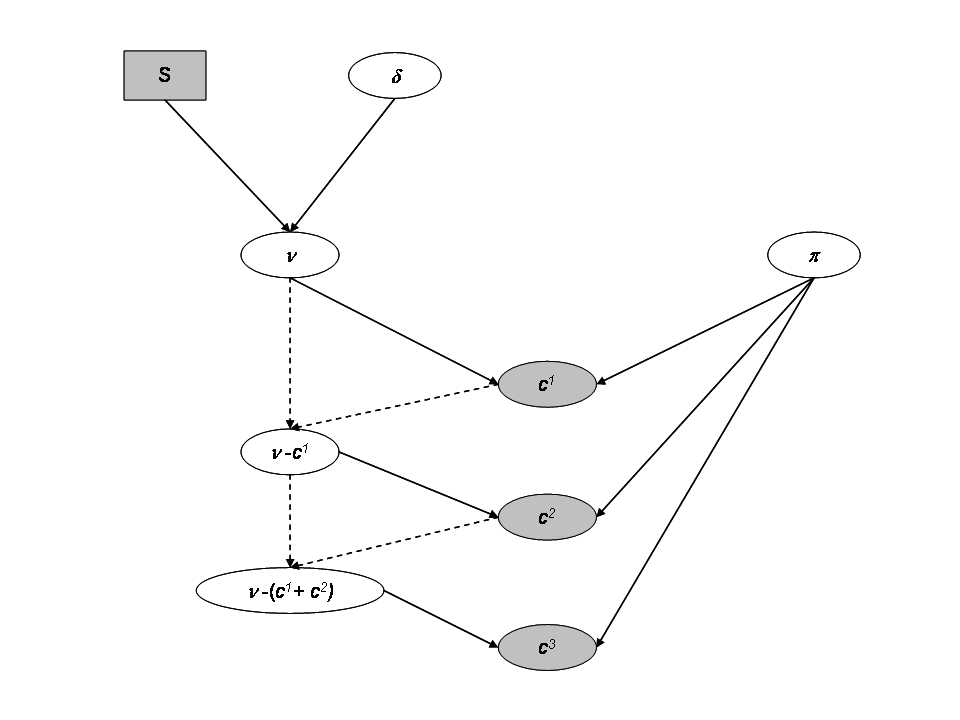Directed Acyclic Graph for a site with the independent model

### Jags implementation and diagnostic for the independtent model

ind_model_str <- "model
{

for (i in 1:I)
{

# Prior
# -----

# Prior on capture efficiency
pi[i] ~ dbeta(1, 1)

# Prior on fish density
delta[i] ~ dgamma(1, 1)

# Number of fish on each site i (surface S[i] in the data set)
mu_nu[i] <- S[i] * delta[i]
nu[i] ~ dpois(mu_nu[i]) T(,500)

# Likelihood
# ----------

C1[i] ~ dbin(pi[i], nu[i])
nur1[i] <- nu[i] - C1[i]
C2[i] ~ dbin(pi[i], nur1[i])
nur2[i] <- nur1[i] - C2[i]
C3[i] ~ dbin(pi[i], nur2[i])

}

}"
set.seed(123)
ind_model <- jags.model(file = textConnection(ind_model_str),
data = SucRemNivelle,
#   inits = replicate(3, ind_init, simplify = FALSE),
n.chains = 3)
#> Warning in jags.model(file = textConnection(ind_model_str), data =
#> SucRemNivelle, : Unused variable "AI" in data
#> Warning in jags.model(file = textConnection(ind_model_str), data =
#> SucRemNivelle, : Unused variable "I_pred" in data
#> Warning in jags.model(file = textConnection(ind_model_str), data =
#> SucRemNivelle, : Unused variable "AI_pred" in data
#> Compiling model graph
#>    Resolving undeclared variables
#>    Allocating nodes
#> Graph information:
#>    Observed stochastic nodes: 74
#>    Unobserved stochastic nodes: 124
#>    Total graph size: 333
#>
#> Initializing model

# Inferences
update(ind_model, n.iter = 10000)
ind_posterior_sample <- coda.samples(ind_model,
variable.names = c("pi", "delta", "nu"),
n.iter = 10000,
thin = 10)
diagnostic <- summarise_draws(as_draws_df(ind_posterior_sample),
default_convergence_measures())
ggplot(diagnostic, aes(x = rhat)) +
geom_histogram() +
theme_classic(base_size = 15)
#> stat_bin() using bins = 30. Pick better value with binwidth.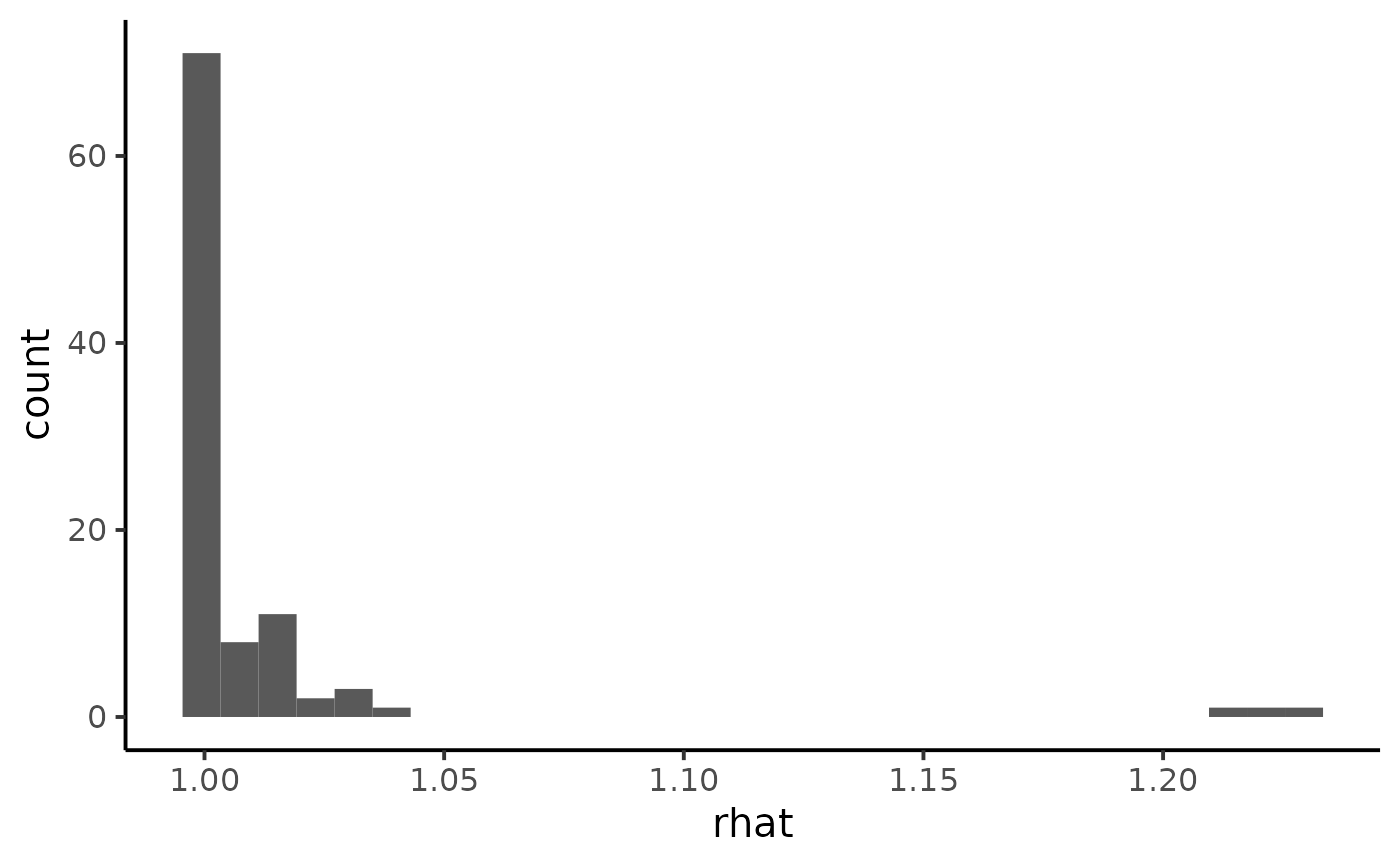ggplot(diagnostic, aes(x = ess_bulk)) +
geom_histogram() +
theme_classic(base_size = 15)
#> stat_bin() using bins = 30. Pick better value with binwidth.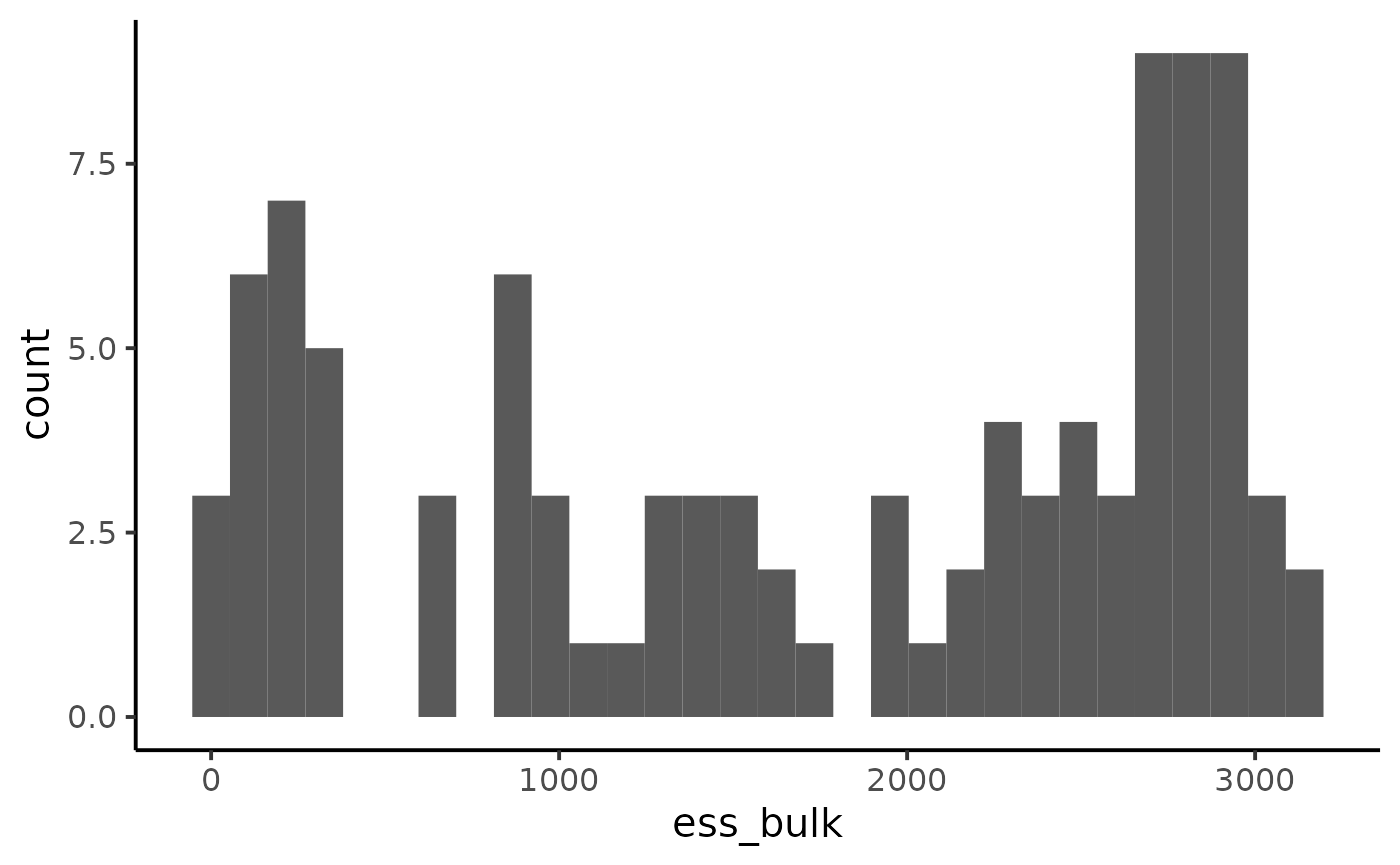We notice some convergence issues on sites with insufficient information (e.g. sites number 5, 10 or 26).

mcmc_trace(ind_posterior_sample, pars = c("delta", "pi", "delta", "pi"))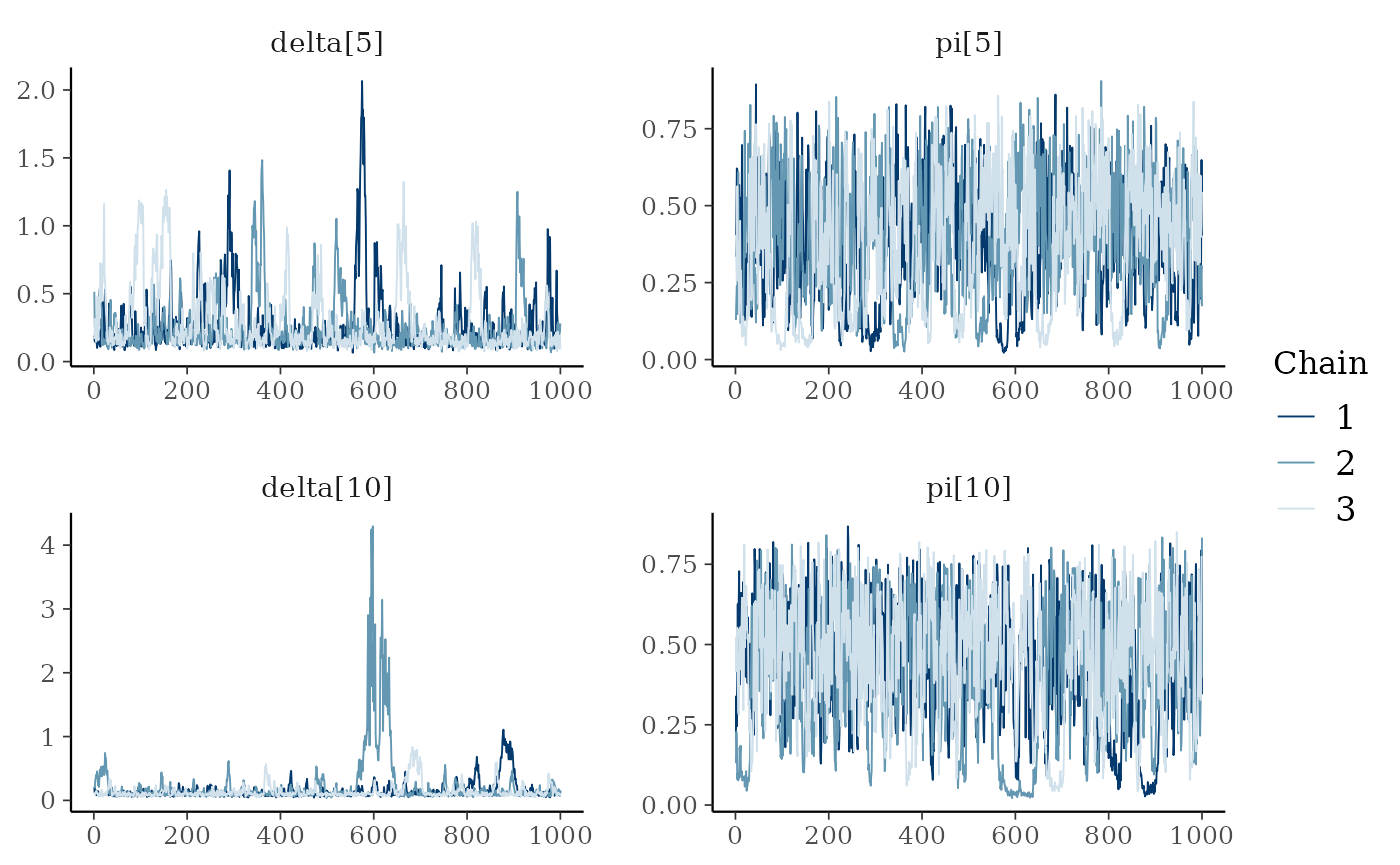dfw_ind <- extract_wider(ind_posterior_sample)

## Hierarchical modeling

We add a hierarchical additional layer that this the experiments together. We do so by adding prior distribution to $$a$$, $$b$$, $$c$$ and $$d$$. For the parameters $$(a, b)$$ we use a simple transformation by considering:

• $$\mu_{a,b} = \frac{a}{a+b}$$ with $$\mu_{a,b} \sim Beta(1.5,1.5)$$, which is the mean of the Beta distribution.
• $$u_{a,b} = a+b$$ with $$\log(u_{a,b}) \sim Uniform(0,10)$$ can be interpreted as a prior sample size that scales the variance of the Beta prior distribution.

We follow the same line of reasoning, a change of variable for parameters $$c$$ and $$d$$ with mean $$\mu_{\delta} = \frac{c}{d}$$ and standard deviation $$\sigma_{\delta} = \frac{\sqrt{c}}{d}$$. Prior knowledge about juvenile salmon density suggests and informative prior for $$\mu_{\delta}$$ and a diffuse prior for $$\sigma_{\delta}$$:

• $$\mu_{\delta} \sim Gamma(1,1)$$
• $$\sigma_{\delta} \sim Gamma(1, 10^{-3})$$.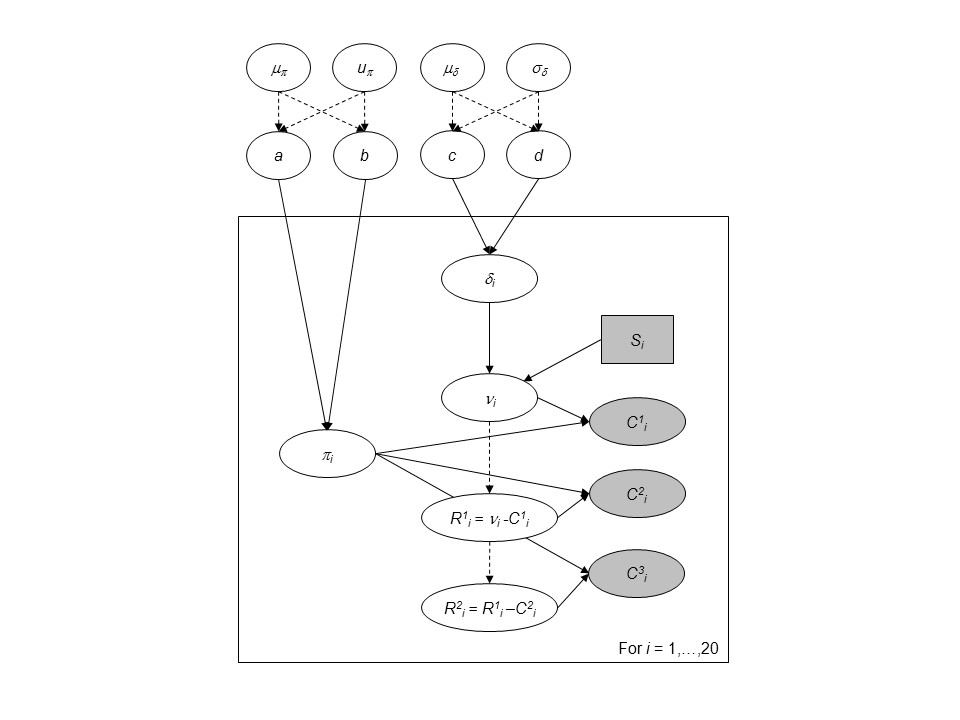Directed Acyclic Graph of the hierarchical model for successive removals.

### Jags implementation and diagnostic for the hierarchical model

hier_model_str <- "model
{

# Priors
# ------

# Hyperprior for the trapping efficiency
mu_p ~ dbeta(1.5 ,1.5)
log_u_p ~ dunif(0,10)
u_p <- exp(log_u_p)

a <- mu_p * u_p
b <- (1-mu_p) * u_p

# Compute standard deviation of p
var_p <- mu_p*(1-mu_p)*(1/(u_p+1))
sd_p <- sqrt(var_p)

# Hyperpriror for the density
mu_d ~ dgamma(1,1)
sd_d ~ dgamma(1,0.001)

# Parameterization of the Gamma distribution
c <- pow(mu_d,2) / pow(sd_d,2)
d <- mu_d / pow(sd_d,2)

for (i in 1:I)
{

# Prior on capture efficiency
pi[i] ~ dbeta(a,b)

# Prior on fish density
delta[i] ~ dgamma(c,d)

# Number of fish (S[i] in the data set)
mu_nu[i] <- S[i] * delta[i]
nu[i] ~ dpois(mu_nu[i]) T(,500)

}

# Likelihood
# ----------

for (i in 1:I)
{
C1[i] ~ dbin(pi[i], nu[i])
nur1[i] <- nu[i] - C1[i]
C2[i] ~ dbin(pi[i], nur1[i])
nur2[i] <- nur1[i] - C2[i]
C3[i] ~ dbin(pi[i], nur2[i])
}

}
"
set.seed(123)
hier_model <- jags.model(
file = textConnection(hier_model_str),
data = SucRemNivelle,
n.chains = 3)
#> Warning in jags.model(file = textConnection(hier_model_str), data =
#> SucRemNivelle, : Unused variable "AI" in data
#> Warning in jags.model(file = textConnection(hier_model_str), data =
#> SucRemNivelle, : Unused variable "I_pred" in data
#> Warning in jags.model(file = textConnection(hier_model_str), data =
#> SucRemNivelle, : Unused variable "AI_pred" in data
#> Compiling model graph
#>    Resolving undeclared variables
#>    Allocating nodes
#> Graph information:
#>    Observed stochastic nodes: 74
#>    Unobserved stochastic nodes: 128
#>    Total graph size: 354
#>
#> Initializing model

# Inferences
update(hier_model, n.iter = 10000)
hier_posterior_sample <- coda.samples(
hier_model,
variable.names = c("a", "b", "c", "d", "mu_d", "mu_p",
"pi", "delta", "nu"),
n.iter = 10000,
thin = 10)
summarise_draws(as_draws_df(hier_posterior_sample),
default_convergence_measures()) %>%
filter(variable %in% c("a", "b", "c", "d", "mu_d", "mu_p")) %>%
knitr::kable()
variable rhat ess_bulk ess_tail
a 1.0000086 2207.324 2654.666
b 1.0001652 2283.140 2688.094
c 1.0000427 2800.646 2710.848
d 0.9999242 2875.790 2885.734
mu_d 0.9999451 2880.455 2597.036
mu_p 1.0006883 2502.721 2700.134
mcmc_trace(hier_posterior_sample,
pars = c("delta", "pi",
"delta", "pi"))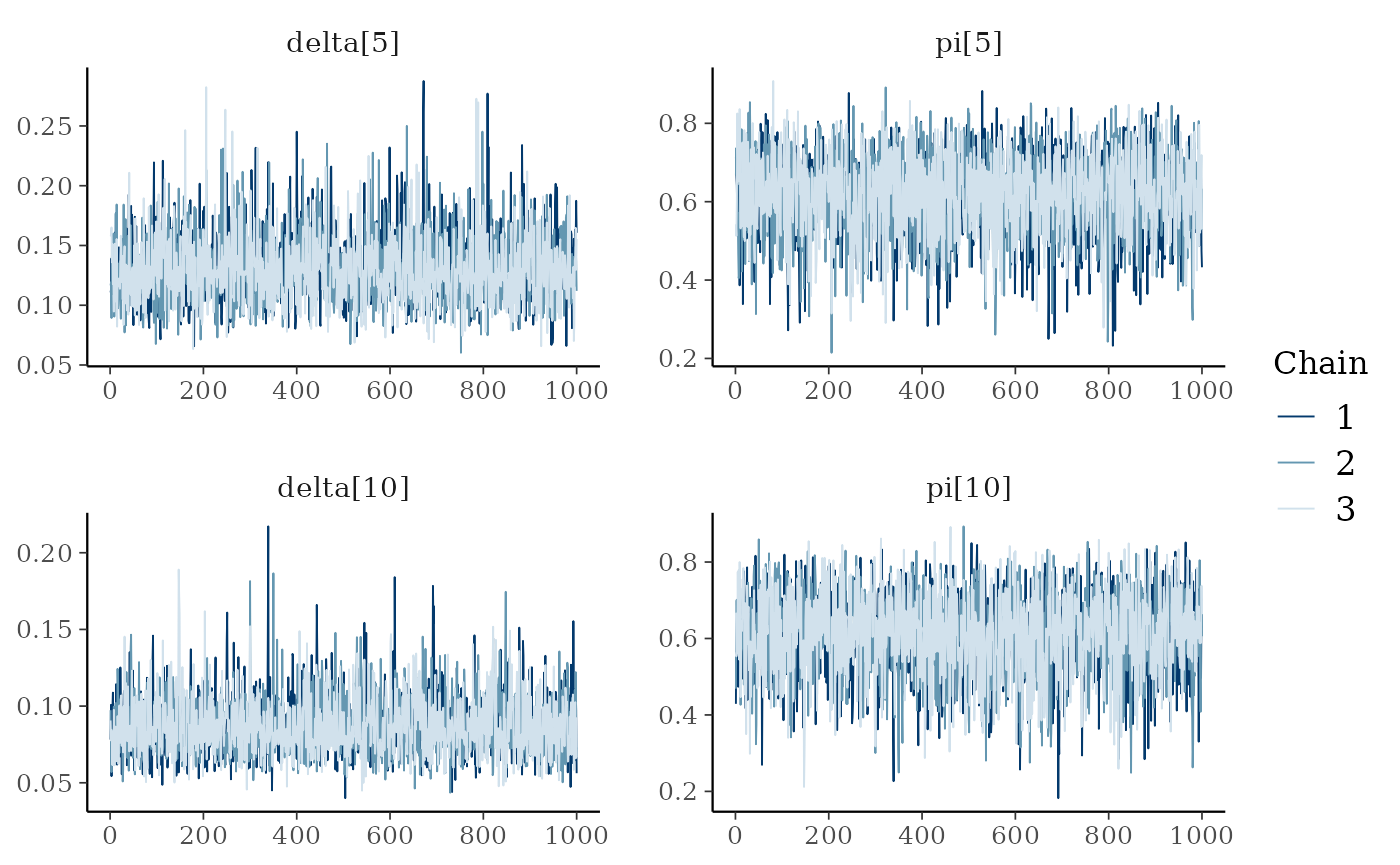### Analysis

dfw_hier <- extract_wider(hier_posterior_sample)

We draw the posterior distribution for the model parameters:

dfw_hier %>%
select("a", "b", "c", "d", "mu_d", "mu_p") %>%
GGally::ggpairs(
columnLabels = c("a", "b", "c", "d", "mu[delta]", "mu[pi]"),
labeller = "label_parsed"
) +
theme_linedraw(base_size = 15L, base_line_size = 0) +
theme(axis.ticks = element_line(size = 15/22))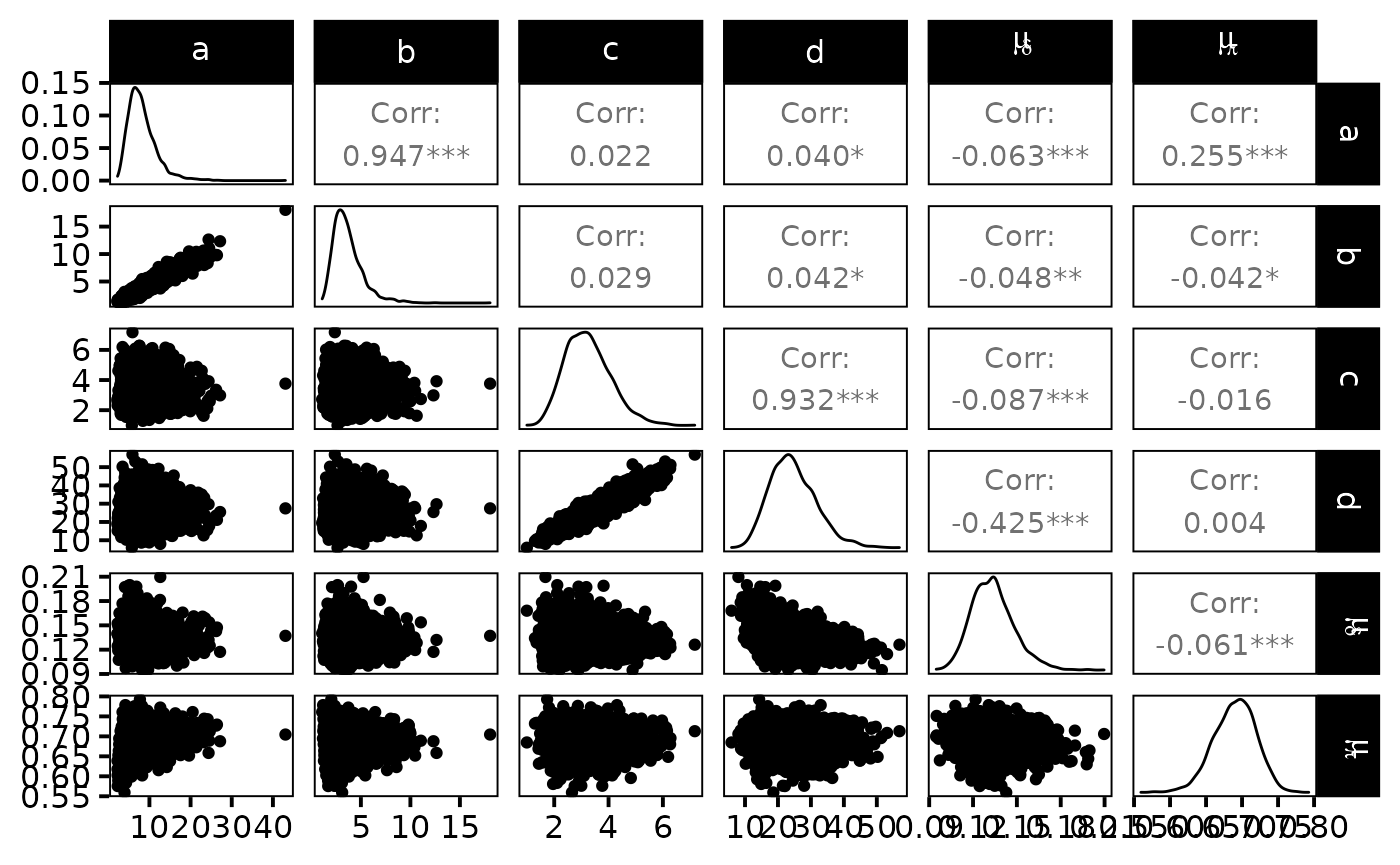The expected efficiency of capture $$\mu_{\pi}$$ lies around $$0.7$$ and the $$\pi$$’s are dispersed among sites approximately like a $$Beta(7,3)$$. We get a good posterior knowledge about the expected density: $$\mu_{\delta}$$ is about $$13$$ individuals per 100$$m^{2}$$. In addition, one obtains a rather small standard deviation $$\sigma_{\delta}$$ of fish densities among sites but, locally, the densities $$\delta_{i}$$ ’s are generally not precisely estimated.

The hierarchical model borrows strength from neighbors. Consequently the problem of banana-shaped joint distribution between $$\pi$$ and $$\delta$$ is reduced when recourse is made to hierarchical modeling. As an example, when considered solely, site 5 and 10 exhibit poor determination of $$\pi$$ and $$\delta$$ as shown in the figures below. The data at site 5 or 10 alone are insufficient to opt for a large capture efficiency $$\pi$$ associated a small population size or for a small capture efficiency with a high local population density $$\delta$$. Under the hierarchical model though, sufficient transfer of information between sites helps specify the probable joint domain where $$\pi$$ and $$\delta$$ are to be expected.

p <- dfw_ind %>%
select(pi.5, delta.5) %>%
ggplot(aes(delta.5, pi.5)) +
geom_point(col = "red") +
geom_density_2d(col = "black") +
theme_bw(base_size = 15L, base_line_size = 0) +
ylim(c(0,1)) +
theme(legend.position="none")+
labs(x=expression(delta),
y=expression(pi),
title="Fish density vs Capture efficiency at site 5",
subtitle = "Independent model")
ggExtra::ggMarginal(p, type = "densigram", bins = 40)
#> Warning: Ignoring unknown parameters: bins
#> Ignoring unknown parameters: bins
#> Ignoring unknown parameters: bins
#> Ignoring unknown parameters: bins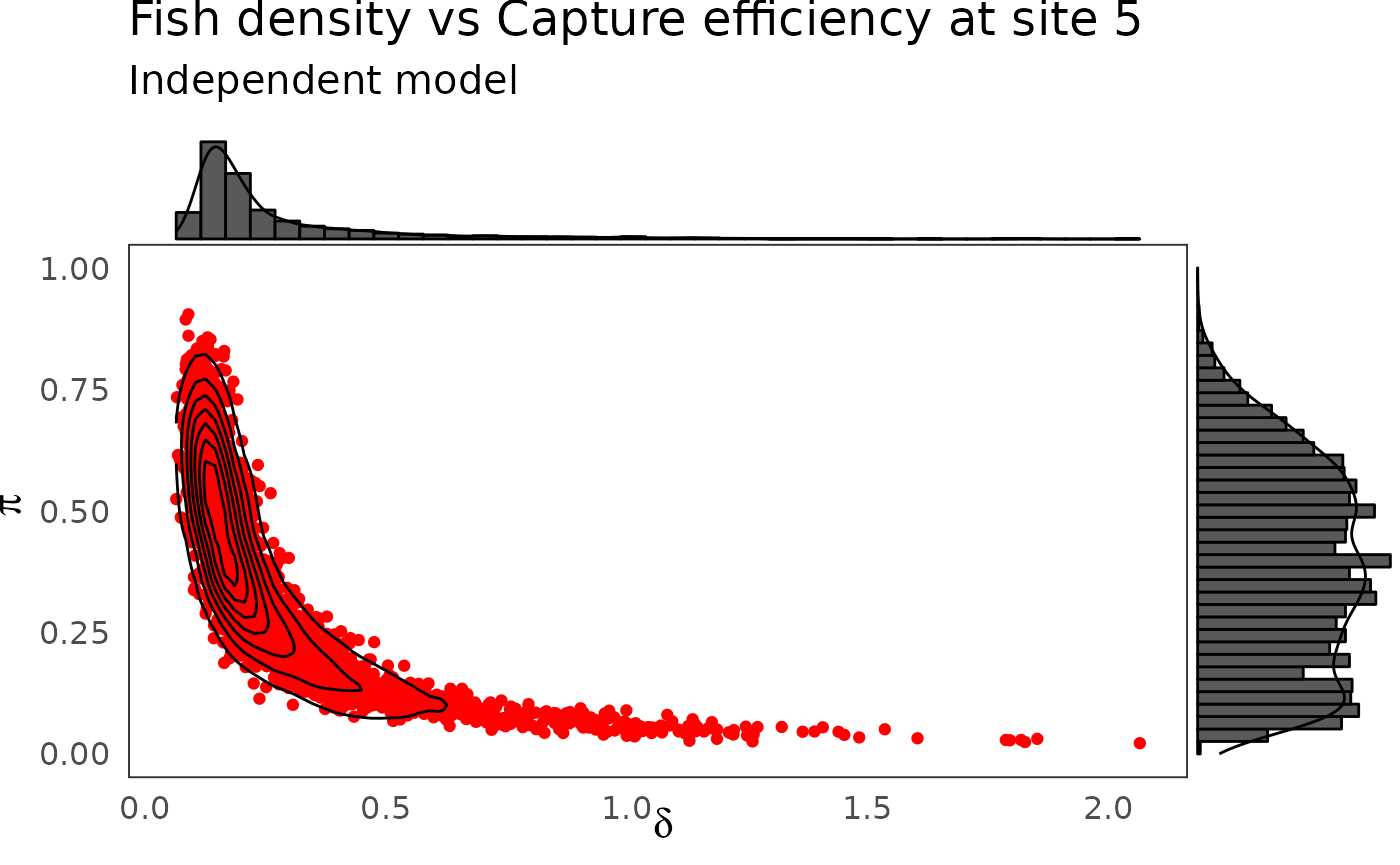p <- dfw_ind %>%
select(pi.10, delta.10) %>%
ggplot(aes(delta.10, pi.10)) +
geom_point(col = "red") +
geom_density_2d(col = "black") +
theme_bw(base_size = 15L, base_line_size = 0) +
ylim(c(0,1)) +
theme(legend.position="none")+
labs(x=expression(delta),
y=expression(pi),
title="Fish density vs Capture efficiency at site 10",
subtitle = "Independent model")
ggExtra::ggMarginal(p, type = "densigram", bins = 40)
#> Warning: Ignoring unknown parameters: bins
#> Ignoring unknown parameters: bins
#> Ignoring unknown parameters: bins
#> Ignoring unknown parameters: bins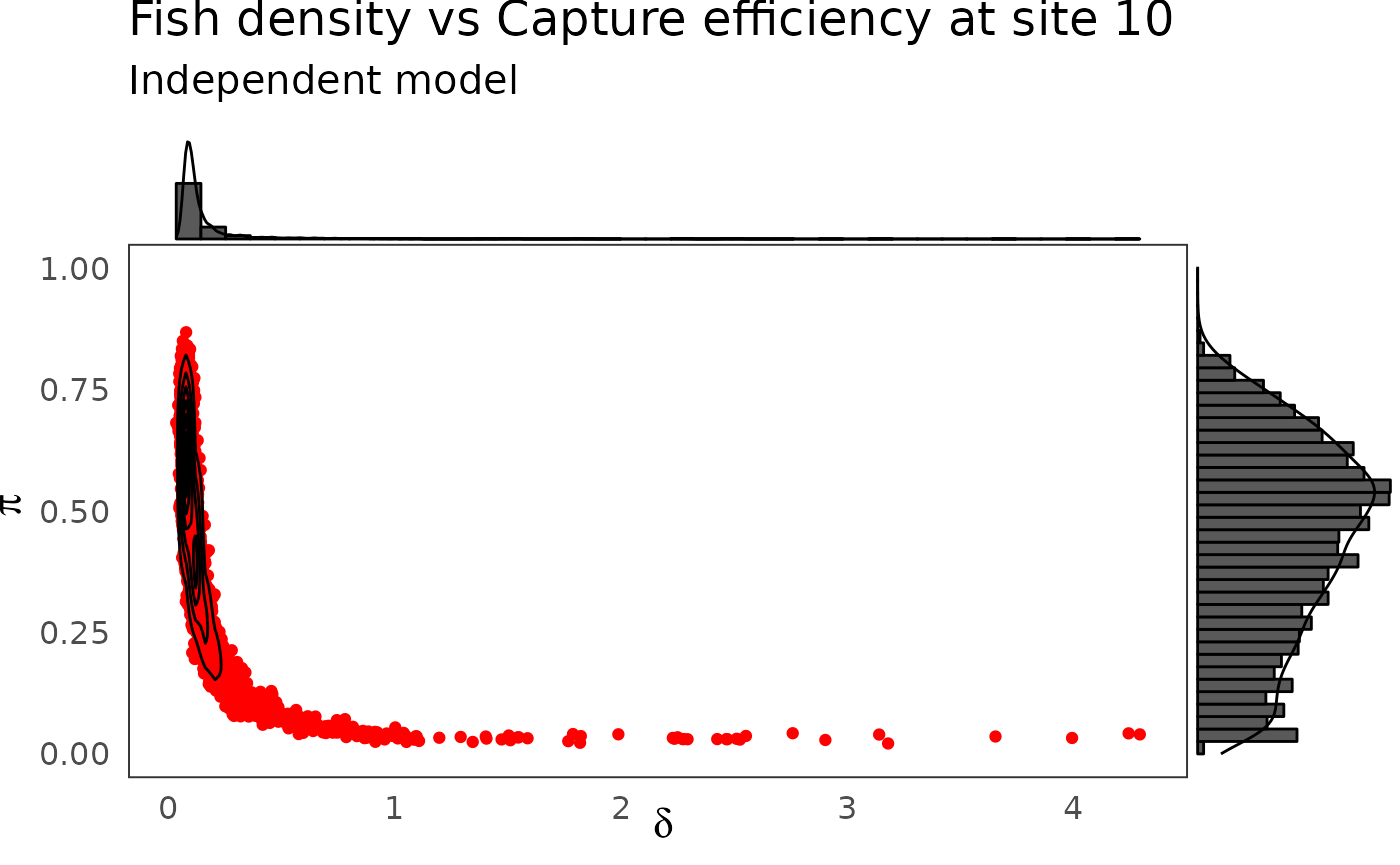phier <- dfw_hier %>%
select(pi.5, delta.5) %>%
ggplot(aes(delta.5, pi.5)) +
geom_point(col = "red") +
geom_density_2d(col = "black") +
ylim(c(0,1)) + xlim(c(0,0.3)) +
theme_bw(base_size = 15L, base_line_size = 0) +
theme(legend.position="none")+
labs(x=expression(delta),
y=expression(pi),title="Fish density vs Capture efficiency at site 5",
subtitle = "Hierarchical model")
ggExtra::ggMarginal(phier, type = "densigram", bins = 40)
#> Warning: Ignoring unknown parameters: bins
#> Ignoring unknown parameters: bins
#> Ignoring unknown parameters: bins
#> Ignoring unknown parameters: bins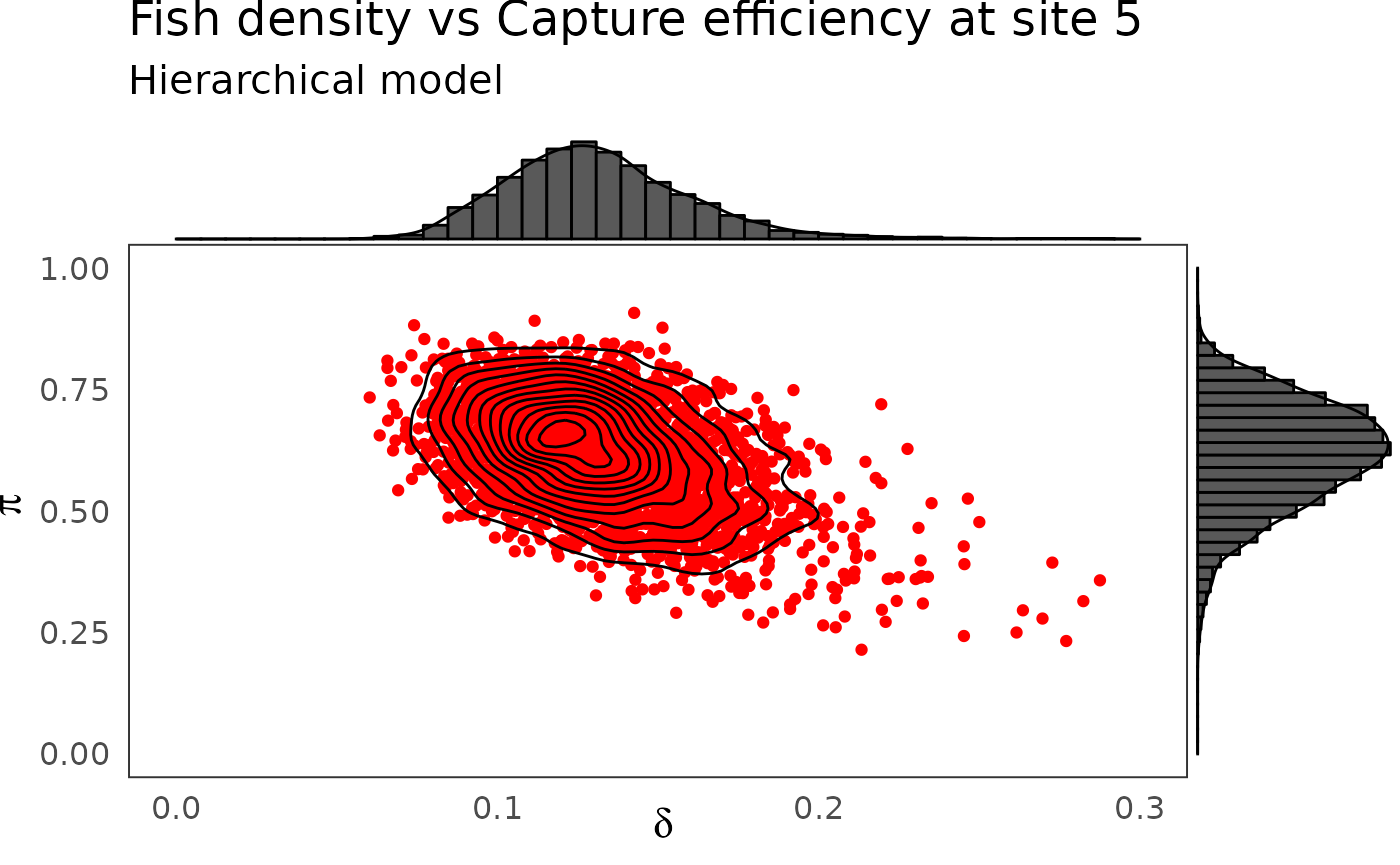phier <- dfw_hier %>%
select(pi.10, delta.10) %>%
ggplot(aes(delta.10, pi.10)) +
geom_point(col = "red") +
geom_density_2d(col = "black") +
ylim(c(0,1)) + xlim(c(0,0.3)) +
theme_bw(base_size = 15L, base_line_size = 0) +
theme(legend.position="none")+
labs(x=expression(delta),
y=expression(pi),
title="Fish density vs Capture efficiency at site 10",
subtitle = "Hierarchical model")
ggExtra::ggMarginal(phier, type = "densigram", bins = 40)
#> Warning: Ignoring unknown parameters: bins
#> Ignoring unknown parameters: bins
#> Ignoring unknown parameters: bins
#> Ignoring unknown parameters: bins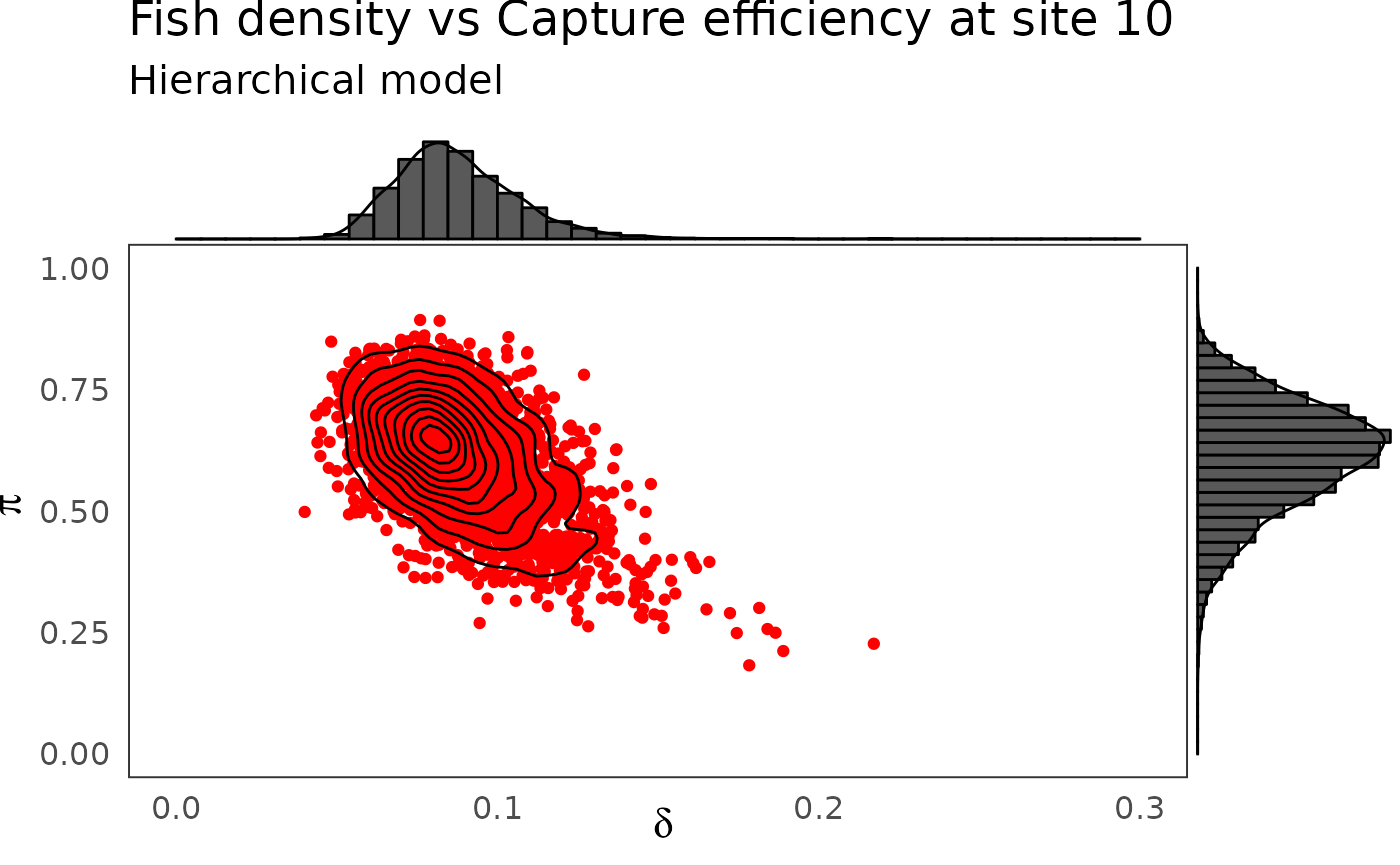## Combining successive removals and a rapid sampling technique

Removal sampling by electric fishing is time and manpower consuming, because it is difficult to store the removed fish and to go thrice in the field for a large number of sites. To increase the number of sites sampled with limited budget and diminishing human resources, recourse can be made to rapid electric fishing assessment techniques. A 5-mn sampling technique requires a single-timed sample for each site under a lighter protocol but only provides relative abundance measures. To be used for absolute population abundance estimation, abundance indices ($$AI$$) need ¨to be first calibrated with another method of population size estimation.

The $$AI$$’s performed on the $$33$$ site of the dataset:

knitr::kable(tibble(Site = 1:SucRemNivelle$I, AI = SucRemNivelle$AI))
Site AI
1 6
2 17
3 12
4 17
5 11
6 22
7 16
8 25
9 25
10 15
11 11
12 4
13 10
14 6
15 9
16 24
17 13
18 16
19 14
20 10
21 25
22 4
23 NA
24 24
25 NA
26 63
27 NA
28 NA
29 21
30 41
31 44
32 NA
33 5

To perform such a calibration in a consistent Bayesian framework, we need to model jointly successive removals and $$AI$$’s. There is a strong link between the observed abundance indices and the mean of the posterior pdf of $$\delta$$ that we take as an estimate of the fish density from each site.

dfl_hier <- extract_longer(hier_posterior_sample) %>% mutate(Model = "Hierarchical")
df_delta_AI  <- dfl_hier %>%
filter(str_detect(parameter, "^delta\\.")) %>%
rename("delta" = value) %>%
mutate(Site = as_factor(str_sub(parameter, 7))) %>%
group_by(Site) %>%
summarise(mean_delta = mean(delta)) %>%
mutate(abundance = SucRemNivelleAI) df_delta_AI %>% ggplot(aes(x = abundance, y = mean_delta)) + ylim(c(0, .4)) + xlim(c(0, 70)) + geom_point() + xlab("Abundance indices") + ylab("Estimated fish density") + theme_classic(base_size = 15L) + labs(caption = "Posterior means of Salmon juvenile density (under hierarchical bayesian model) versus observed Abundance Indices.") #> Warning: Removed 5 rows containing missing values (geom_point).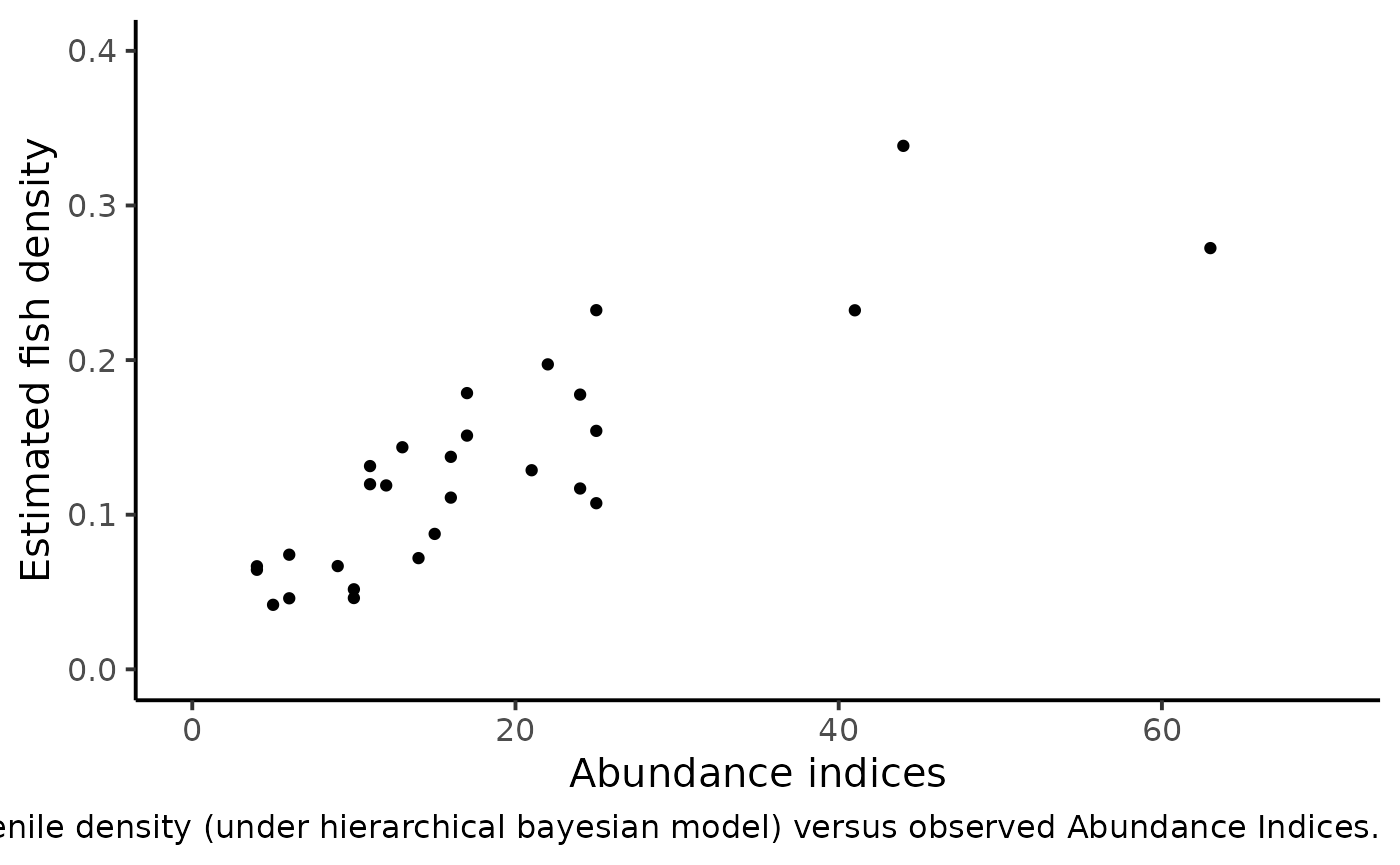We can figure out a linear trend but with an increasing dispersion. Conversely to the homoscedastic behavior of the linear regression model, the variance should depend on the mean. Keeping these clues in mind, we relate the vector of Salmon juveniles’ density $$\delta_{i}$$’s to the vector of abundance indices $$AI_{i}$$’s as follows: • As we are counting events, we hypothesize for each site $$i$$, a Poisson distribution for AI around a mean value $$\mu_{AI}:$$ $AI_{i}~\sim~Poisson(\mu_{AI_{i}})$ • In turn, this true mean value $$\mu_{AI}$$ will be drawn from a Gamma distribution. $\mu_{AI_{i}} ~ \sim~ Gamma(\alpha_{AI_{i}},\beta_{AI_{i}})$ Such a choice can be hypothesized for two reasons: • The convolution of a Poisson by a Gamma distribution makes a negative Binomial pdf, a common model for overdispersion in a counting experiment; • By setting $$\beta_{AI_{i}}=f,\ \alpha_{AI{i}}=k\times f\times\delta_{i}$$, one can describe a variance increasing (linearly) with the mean since: \left\{ \begin{aligned} & \mathbb{E}(\mu_{AI_{i}}) = \frac{\alpha_{AI_{i}}}{\beta_{AI_{i}}}% = k\delta_{i}\\ & \mathbb{V}(\mu_{AI_{i}}) = \frac{\alpha_{AI_{i}}}{\beta_{AI_{i}}^{2}}% = \frac{ \mathbb{E} (\mu_{AI_{i}}) }{f} \end{aligned} \right. \end{enumerate} The additional parameters $$k$$ and $$f$$ must be assigned a prior pdf. Parameter $$k$$ is given a flat noninformative gamma prior: $k~\sim~Gamma(1,10^{-3})$ It is convenient to work with $$\phi$$ such that $$F=\frac{\phi}{1-\phi}$$. $$\phi$$ is the probability parameter from the Negative Binomial and we naturally opt for a uniform pdf: $\phi ~ \sim~Beta(1,1)$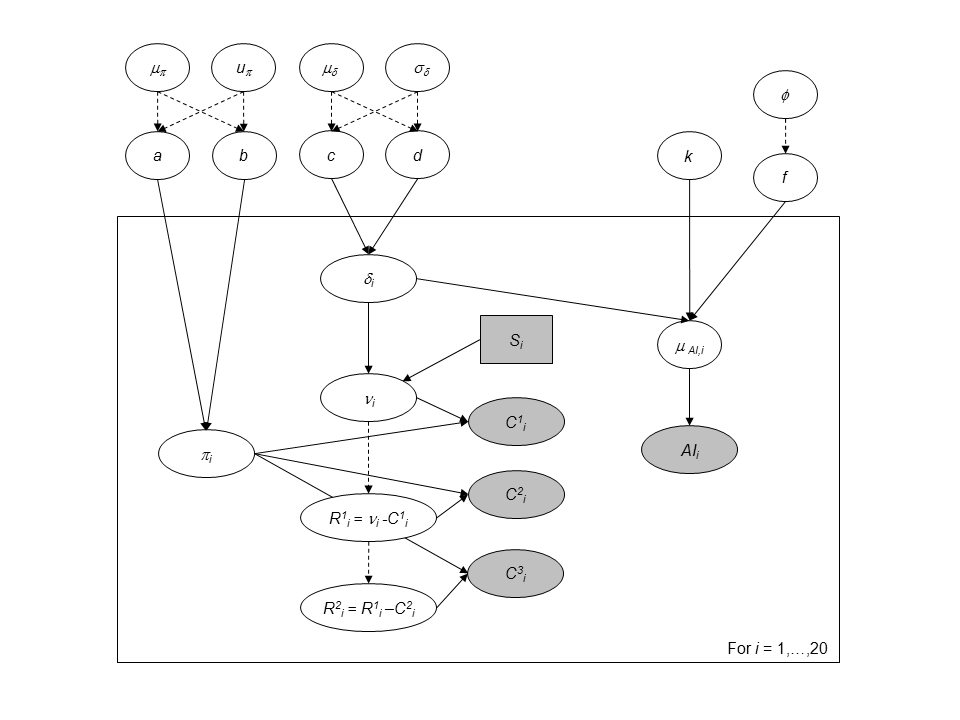Directed Acyclic Graph of the hierarchical model for successive removals including abundance indices as an additional source of information for the 0+ juveniles density. ai_model_str <- "model { # Priors # ------ # Hyperprior for the trapping efficiency mu_p ~ dbeta(1,1) log_u_p ~ dunif(0,10) u_p <- exp(log_u_p) a <- mu_p * u_p b <- (1-mu_p) * u_p # Compute standard deviation of p var_p <- mu_p*(1-mu_p)*(1/(u_p+1)) sd_p <- sqrt(var_p) # Hyperpriror for the density mu_d ~ dgamma(1,1) sd_d ~ dgamma(1,0.001) # Parameterization of the Gamma distribution c <- pow(mu_d,2) / pow(sd_d,2) d <- mu_d / pow(sd_d,2) for (i in 1:I) { # Prior on capture efficiency pi[i] ~ dbeta(a,b) # Prior on fish density delta[i] ~ dgamma(c,d) # Number of fish (S[i] in the data set) mu_nu[i] <- S[i] * delta[i] nu[i] ~ dpois(mu_nu[i]) T(,500) } # Hyperpriors for the mean abundance index k ~ dgamma(1, 0.001) phi ~ dbeta(1, 1) f <- phi/(1-phi) # Priors for the Abundance index for (i in 1:I) { alpha_AI[i] <- k*f*delta[i] mu_AI[i] ~ dgamma(alpha_AI[i], f) } # Likelihood # ---------- for (i in 1:I) { C1[i] ~ dbin(pi[i], nu[i]) nur1[i] <- nu[i] - C1[i] C2[i] ~ dbin(pi[i], nur1[i]) nur2[i] <- nur1[i] - C2[i] C3[i] ~ dbin(pi[i], nur2[i]) AI[i] ~ dpois(mu_AI[i]) } # Predictive distribution of the density conditionnally upon # observed abundance indices for (i in 1:I_pred) { AI_pred[i] ~ dpois(mu_AI_pred[i]) mu_AI_pred[i] ~ dgamma(alpha_AI_pred[i], f) alpha_AI_pred[i] <- k * delta_pred[i] * f delta_pred[i] ~ dgamma(c,d) # Link with observed abundance indices } } " set.seed(123) ai_model <- jags.model(file = textConnection(ai_model_str), data =SucRemNivelle,#c(dataListvalue, data_AI),
n.chains = 3)
#> Compiling model graph
#>    Resolving undeclared variables
#>    Allocating nodes
#> Graph information:
#>    Observed stochastic nodes: 167
#>    Unobserved stochastic nodes: 298
#>    Total graph size: 717
#>
#> Initializing model

# Inferences
update(ai_model, n.iter = 10000)
ai_posterior_sample <- coda.samples(
ai_model,
variable.names = c("a", "b", "c", "d", "mu_d", "mu_p", "sd_d", "sd_p",
"pi", "delta", "nu", "phi", "k", "f", "mu_AI",
"delta_pred", "mu_AI_pred"),
n.iter = 10000,
thin = 10)
summarise_draws(as_draws_df(ai_posterior_sample),
default_convergence_measures()) %>%
filter(variable %in% c("a", "b", "c", "d",
"mu_d", "mu_p", "sigma_d", "sigma_p",
"phi", "k", "mu_AI")) %>%
knitr::kable()
variable rhat ess_bulk ess_tail
a 0.9998840 2029.4588 2781.9576
b 1.0002102 2294.4066 2829.9883
c 1.0042604 1116.3178 2283.0722
d 1.0094108 723.2803 1751.8044
k 1.0139487 448.7799 790.3346
mu_d 1.0113785 666.4471 1436.7747
mu_p 0.9993467 2560.4025 2899.8039
phi 1.0154339 335.2859 412.8917
mcmc_trace(ai_posterior_sample,
pars = c("delta", "pi",
"delta", "pi"))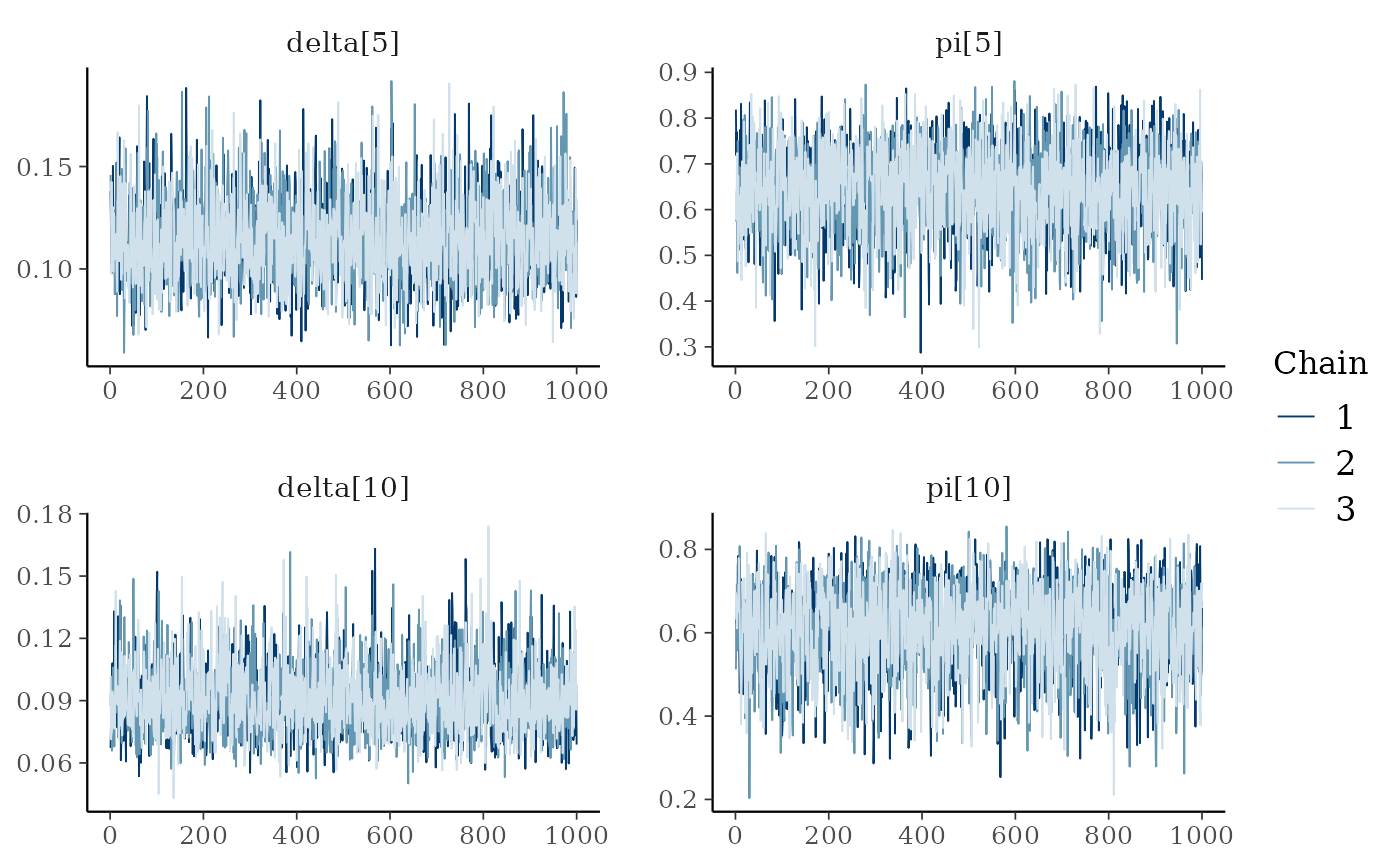### Results and analysis

dfw_ai <- extract_wider(ai_posterior_sample)
dfw_ai %>%
select("mu_d", "sd_d", "mu_p",  "sd_p", "phi", "k") %>%
GGally::ggpairs(
columnLabels = c("mu[delta]", "sigma[delta]", "mu[pi]",  "sigma[pi]", "phi", "k"),
labeller = "label_parsed",
lower = list(continuous = wrap("points", alpha = 0.3,size = .5))
) +
theme_linedraw(base_size = 15L, base_line_size = 0) +
theme(axis.ticks = element_line(size = 15/22)) +
labs(caption = "Posterior distributions of parameters for the full AI + successive removal model.")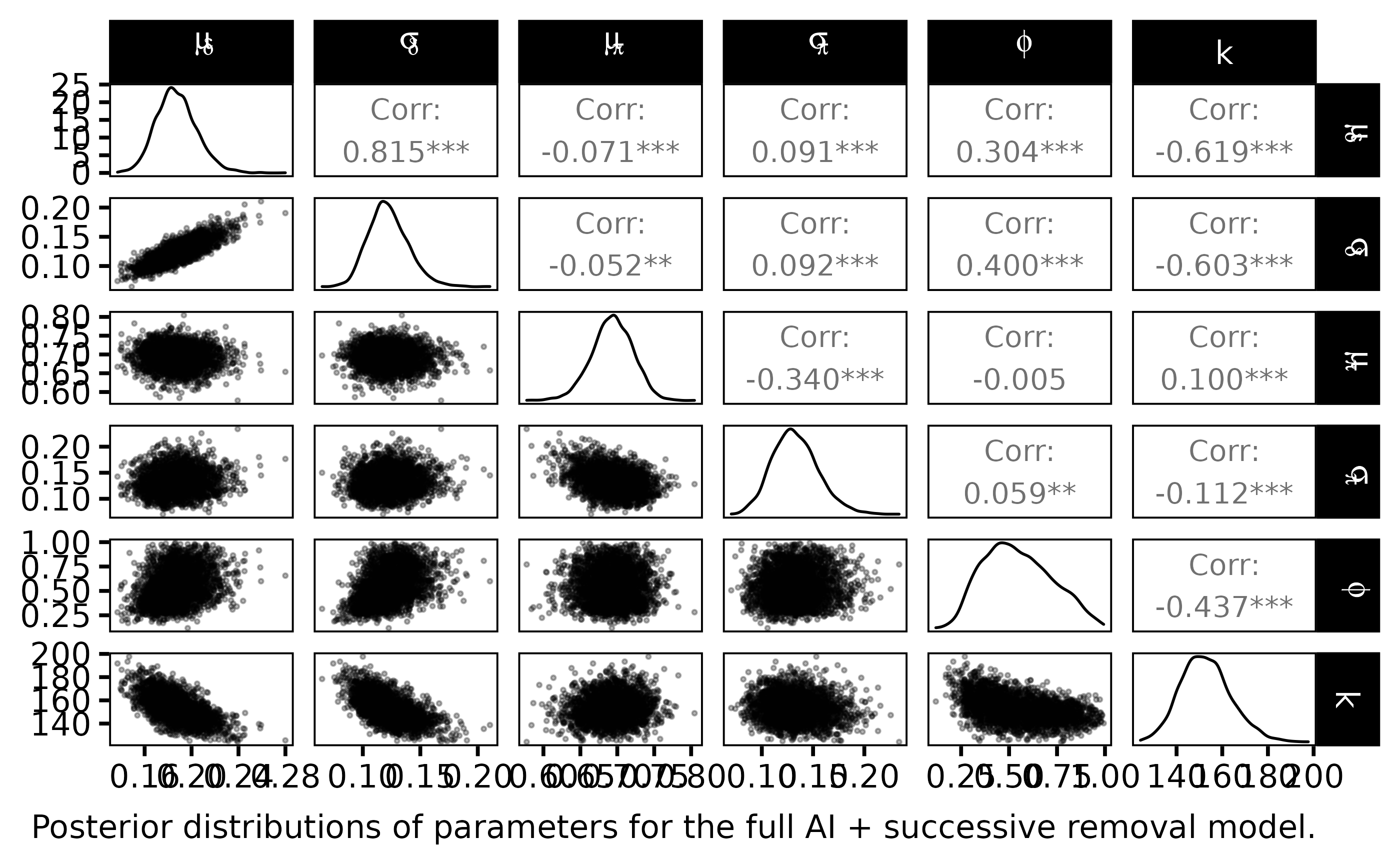The statistical Bayesian learning machinery provides informative posterior distribution for $$\phi$$ and $$k$$:

• $$\phi$$ is far from uniform, its posterior pdf varies around $$0.65$$
• $$k$$ varies close to $$150$$ with a small standard deviation
dfl_ind <- extract_longer(ind_posterior_sample) %>%  mutate(Model = "Independent")
dfl_ai <- extract_longer(ai_posterior_sample) %>%  mutate(Model = "Abundance Index")
bind_rows(dfl_hier, dfl_ind, dfl_ai) %>%
filter(str_detect(parameter, "^delta\\.")) %>%
rename("delta" = value) %>%
mutate(Site = as_factor(str_sub(parameter, 7))) %>%  # 7 = length("delta.") + 1
ggplot() +
aes(x = Site, y = delta, fill = Model ) +
geom_boxplot(outlier.shape = NA, outlier.size = .25) +
scale_x_discrete(guide = guide_axis(angle = 90)) +
ylim(c(0,0.75)) +
scale_fill_manual(values = c("gray10", "gray50", "gray90")) +
ylab("Fish density") +
theme_classic(base_size = 15L) +
labs(caption = expression("Marginal posterior distributions of salmon densities delta for each site index."))
#> Warning: Removed 2188 rows containing non-finite values (stat_boxplot).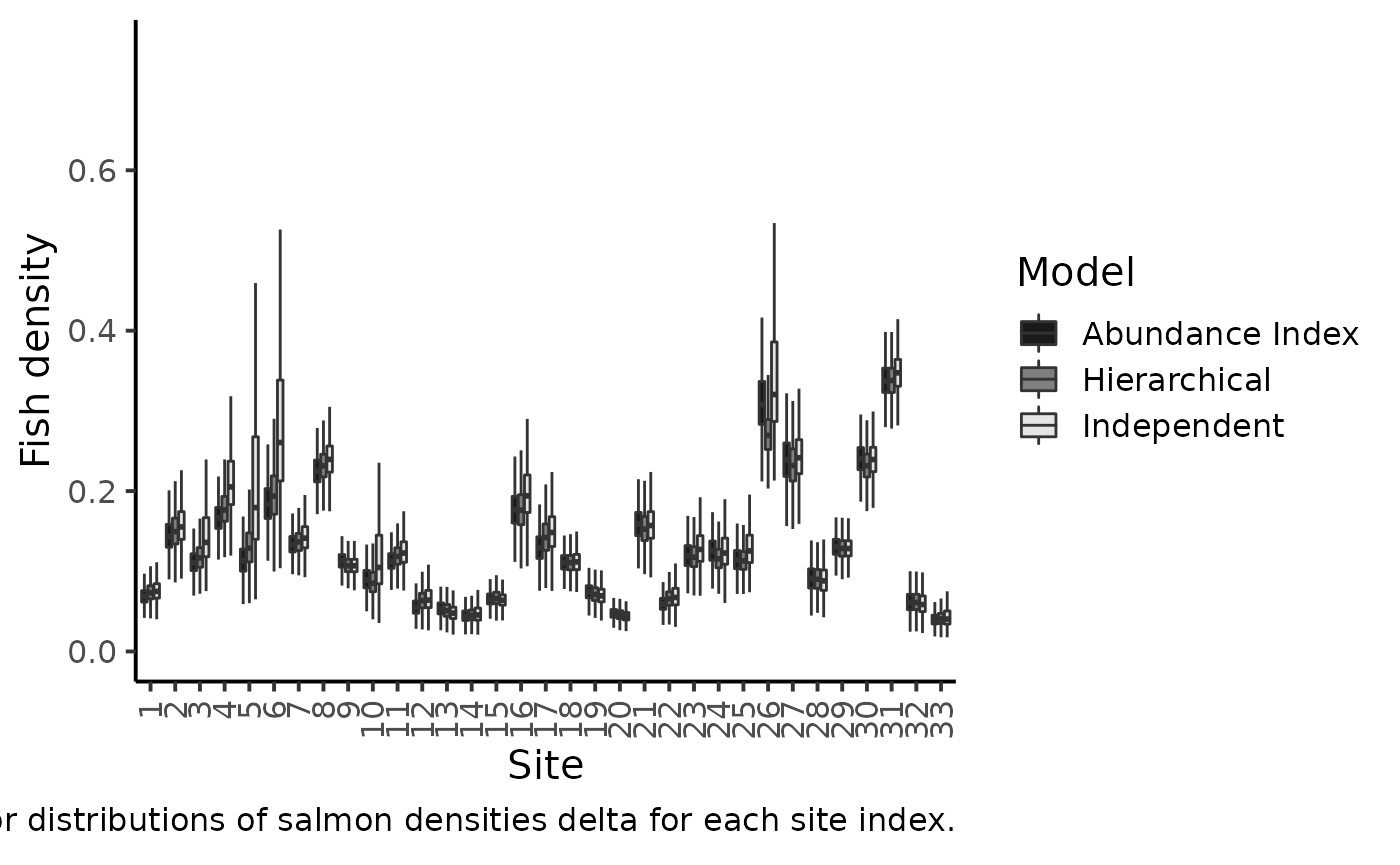The figure makes clear the shrinkage effect due to hierarchical modeling: with the hierarchical model, the densities are less dispersed around the grand mean. Sharing information between sites allows for more precise estimations of the local fish densities. The black boxplotsdepict the posterior pdf of fish density $$\delta$$ when modeling jointly the successive removals and the $$AI$$’s. When compared to the previous model without taking into account the information conveyed by the abundance index (grey boxplots), we see that there is only a small shrinkage effect: although the 50% credible intervals are generally narrower, i.e., we learn something from the $$AI$$ sample but not that markedly. Site $$26$$ bears a noticeable exception that can be explained by contradictory information brought by successive removals and by AI. If we were to trust the successive removal model only, the juvenile density for site $$26$$ should be around $$0.3$$ and, as $$k$$ is close to $$140$$, we should expect a value of $$150\times0.3=45$$ individuals for the abundance index, but we actually observed $$63$$ juveniles in the $$AI$$ data!

dfl_ai %>%
filter(str_detect(parameter, "^delta_pred.")) %>%
mutate(AI = as.numeric(str_sub(parameter, 12))) %>%
rename("delta_pred" = value) %>%
group_by(AI) %>%
summarise(qs = quantile(delta_pred,
c(.1, .25, .5, .75, .9)),
prob = c(.025, .25, .5, .75, .975)) %>%
pivot_wider(names_from = prob, values_from =  qs) %>%
ggplot(aes(x = AI, y = 0.5)) +
geom_ribbon(aes(ymin = 0.025, ymax = 0.975), fill = "gray50") +
geom_ribbon(aes(ymin = 0.25, ymax = 0.75), fill = "gray75") +
geom_line() +
geom_point(data = df_delta_AI,
mapping = aes(x = abundance, y = mean_delta)) +
xlab("Abundance indices") +
ylab("Posterior predictive of fish density") +
theme_classic(base_size = 15L) +
labs(caption = "Predictive posterior distribution of Salmon juvenile density versus Abundance Indices (50% and 95% credible interval)")
#> summarise() has grouped output by 'AI'. You can override using the .groups
#> argument.
#> Warning: Removed 5 rows containing missing values (geom_point).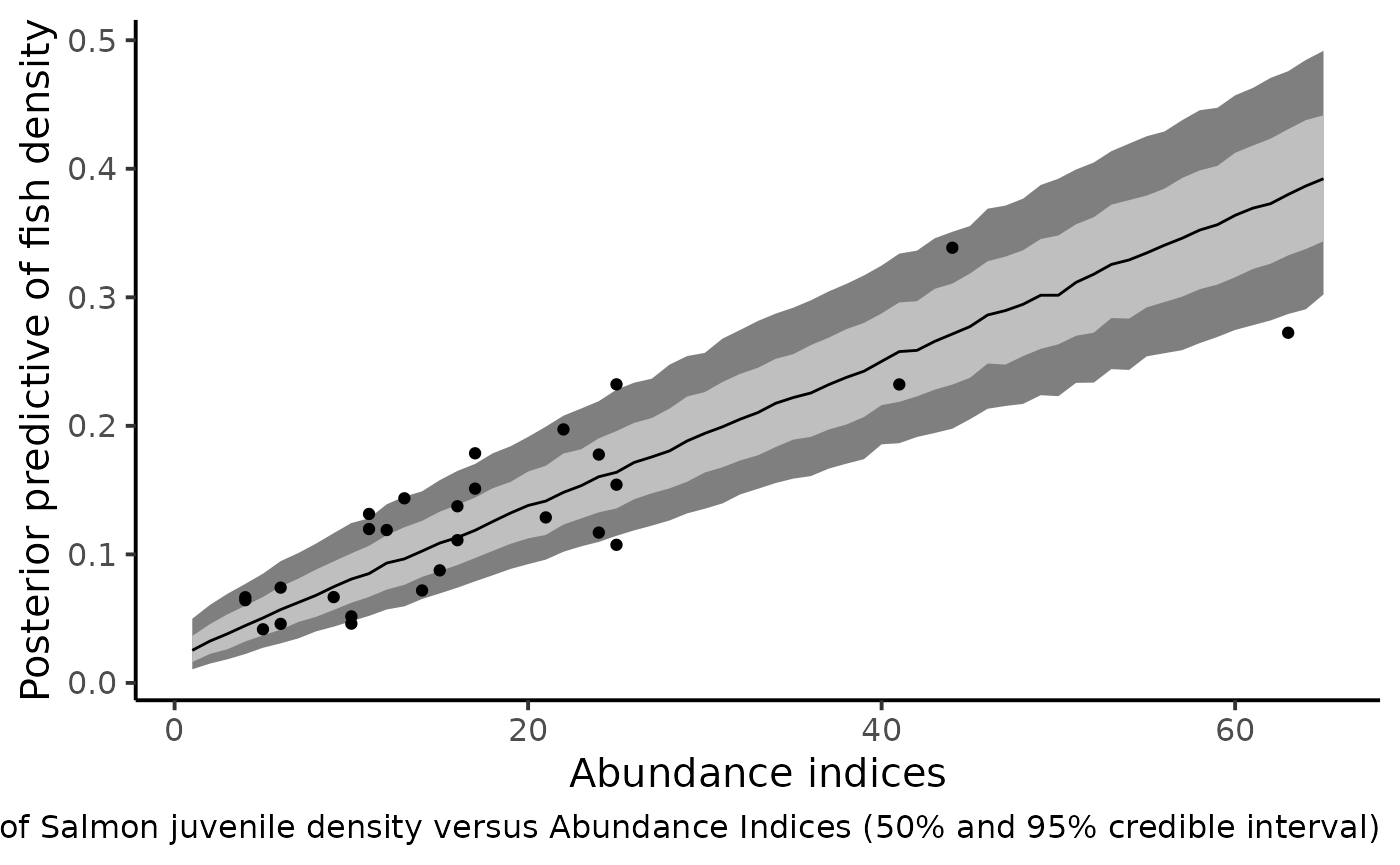The figure shows that the index of abundance alone allows for differentiation between contrasting levels of fish density without recourse to the successive removal technique in the future. Yet fairly imprecise, this rapid sampling technique also provides the corresponding confidence bounds that match the cloud made by the posterior means of Salmon juvenile density (under the HBM model) and the Abundance Indices observed in the dataset.

Parent, E., and E. Prévost. 2013. Introduction to Hierarchical Bayesian Modeling for Ecological Data. CRC press Boca Raton, Florida.